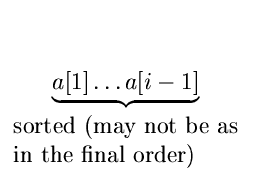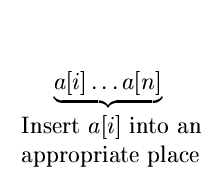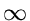Next:9.3 Selection SortUp:9. Sorting MethodsPrevious:9.1 Bubble Sort

# 9.2 Insertion Sort

Here in the ith iteration, the record a[i] is inserted into its proper position in the first i positions.Let us assume, for the sake of convenience, a fictitious record a with key value =.

{

for(i = 2;i < = n;i + +)

{

j = i ;

while (key of a[j] < key of a[j - 1])

{

swap records a[j] and a[j - 1];

j = j - 1

}

}

}

• Exhibits the worst case performance when the initial array is sorted in reverse order.
• Worst case and average case performance is O(n2)

eEL,CSA_Dept,IISc,Bangalore